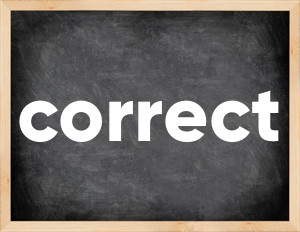# Correct past tenseThe English verb 'correct' is pronounced as [kəˈrekt].
Related to: regular verbs.
3 forms of verb correct: Infinitive (correct), Past Simple - (corrected), Past Participle - (corrected).

## Here are the past tense forms of the verb correct

👉 Forms of verb correct in future and past simple and past participle.
❓ What is the past tense of correct.

## Correct: Past, Present, and Participle Forms

Base Form Past Simple Past Participle
correct [kəˈrekt]

corrected [kəˈrektɪd]

corrected [kəˈrektɪd]

## What are the 2nd and 3rd forms of the verb correct?

🎓 What are the past simple, future simple, present perfect, past perfect, and future perfect forms of the base form (infinitive) 'correct'?

### Learn the three forms of the English verb 'correct'

• the first form (V1) is 'correct' used in present simple and future simple tenses.
• the second form (V2) is 'corrected' used in past simple tense.
• the third form (V3) is 'corrected' used in present perfect and past perfect tenses.

## What are the past tense and past participle of correct?

The past tense and past participle of correct are: correct in past simple is corrected, and past participle is corrected.

### What is the past tense of correct?

The past tense of the verb "correct" is "corrected", and the past participle is "corrected".

### Verb Tenses

Past simple — correct in past simple corrected (V2).
Future simple — correct in future simple is correct (will + V1).
Present Perfect — correct in present perfect tense is corrected (have/has + V3).
Past Perfect — correct in past perfect tense is corrected (had + V3).

### correct regular or irregular verb?

👉 Is 'correct' a regular or irregular verb? The verb 'correct' is regular verb.

## Examples of Verb correct in Sentences

•   It was corrected to 2.5 kg/ha. (Past Simple)
•   These anomalies have not been corrected for 3 years. (Present Perfect)
•   Sorry, I stand corrected. (Present Simple)
•   The errors will be corrected in the book's third edition. (Future Simple)
•   Correct me if I'm wrong. (Present Simple)
•   I hate it when she corrects my pronunciation. (Present Simple)
•   'She's in Britain now.' 'She was,' Lucy corrected him. (Past Simple)
•   Let me correct the article. (Present Simple)
•   He should correct his taste in people. (Present Simple)
•   Why haven't you corrected me? (Present Perfect)

Along with correct, words are popular document and thrust.

Verbs by letter: , , , , , , , , , , , , , , , , , , , , , , , , .